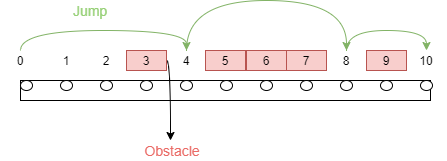6

# Avoiding traps

Difficulty: MEDIUMContributed By
Sounak Majumder
Avg. time to solve
25 min
Success Rate
60%

Problem Statement

#### Find the minimum length of the jump so that we can reach the end of the line avoiding all obstacles.

##### Note:
``````1.The end will be a minimum possible coordinate, greater than the maximum element in the given array of elements.

2.Avoiding obstacles means that we cannot stop at the given coordinates.

3.The elements may not be in sorted order.

4.The last jump can be of any unit, provided it crosses the endpoint.
``````
##### Input format:
``````The first line of input contains an integer ‘T’ denoting the number of test cases.
The next 2 * T lines represent the ‘T’ test cases.

The first line of each test case contains an integer ‘N’, denoting the number of obstacles on the straight line.

The second line of each test case contains an array 'OBSTACLES' of 'N' elements, denoting the obstacles on the straight line.
``````
##### Output format:
``````For each test case, print a single line containing a single integer denoting the minimum length of the jump to reach the end, avoiding all the obstacles.

The output of each test case will be printed in a separate line.
``````
##### Note
``````You don't have to print anything. It has already been taken care of. Just implement the given function.
``````
##### Constraints
``````1 <= T <= 50
1 <= N <= 1000
1 <= OBSTACLES[i] <= 10 ^ 6

Where ‘T’ is the total number of test cases, ‘N’ denotes the number of obstacles on the straight line, and ‘OBSTACLES[i]’ denotes the coordinates of obstacles on the straight line.

Time limit: 1 sec.
``````
##### Sample Input 1:
``````2
5
5 3 6 7 9
5
5 8 9 13 14
``````
##### Sample Output 1:
``````4
6
``````
##### Explanation of sample input 1:
``````Test case 1
````````````Obstacles are at positions 5, 3, 6, 7, 9.
If we take a jump of size 1, it will touch 3 and so it is not viable
For jump size 2, it will touch 6 and so it is discarded.
For jump size 3, it will touch 3 and so it is also discarded.

For jump size 4, we will jump from 0 to 4, then 4 to 8, then 8 to 12. Hence, we reach the end by avoiding all the obstacles.

Test case 2:

Obstacles are at positions 5, 8, 9, 13, 14.
With jump size 6, we will jump from 0 to 6, then 6 to 12, followed by 12 to 18. Hence, we reach the end by avoiding all the obstacles.
``````
##### Sample Input 2:
``````1
6
10 1 2 3 6 9
``````
##### Sample Output 2:
``````4
``````Console